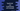# How to use forEach in typescript array## How to use forEach in typescript array:

forEach method is defined in typescript array. It is used to iterate over the items of an array. It can be used with arrays, maps, sets etc.

In this post, we will learn how to use forEach method with examples.

### Definition of forEach:

forEach method is defined as below:

``forEach(callback: (value: number, index: number, array: number[]) => void, thisArg?: any): void``

Here,

• callback is a function that will be called for each element in the array. It accepts three arguments. value is the current value in the array, index is the current index of the value in the array and array is the array whose elements are iterating. The values are optional.
• thisArg is an object to which this keyword can refer to in the callback function.

### Example of forEach:

Let me show you an example of forEach:

``````let givenArr = [1,2,3,4,5,6,7,8]

givenArr.forEach((item) => {
console.log(item);
});``````

In this example, we are iterating through the elements of givenArr using forEach. The callback function is taking only the iterating element.

It will print:

``````1
2
3
4
5
6
7
8``````

We can also read the index of each element as well:

``````let givenArr = [1,2,3,4,5,6,7,8]

givenArr.forEach((item, index) => {
console.log('givenArr['+index+'] = '+ item);
});``````

It will print:

``````givenArr = 1
givenArr = 2
givenArr = 3
givenArr = 4
givenArr = 5
givenArr = 6
givenArr = 7
givenArr = 8``````

### JavaScript conversion:

The above program looks as like below in JavaScript:

``````"use strict";
let givenArr = [1, 2, 3, 4, 5, 6, 7, 8];
givenArr.forEach((item, index) => {
console.log('givenArr[' + index + '] = ' + item);
});``````

### Example of iterating through an array of strings:

We can also iterate through an array of strings using forEach method:

``````let givenArray = ['one', 'two', 'three', 'four'];

givenArray.forEach((item) => {
console.log(item);
});``````